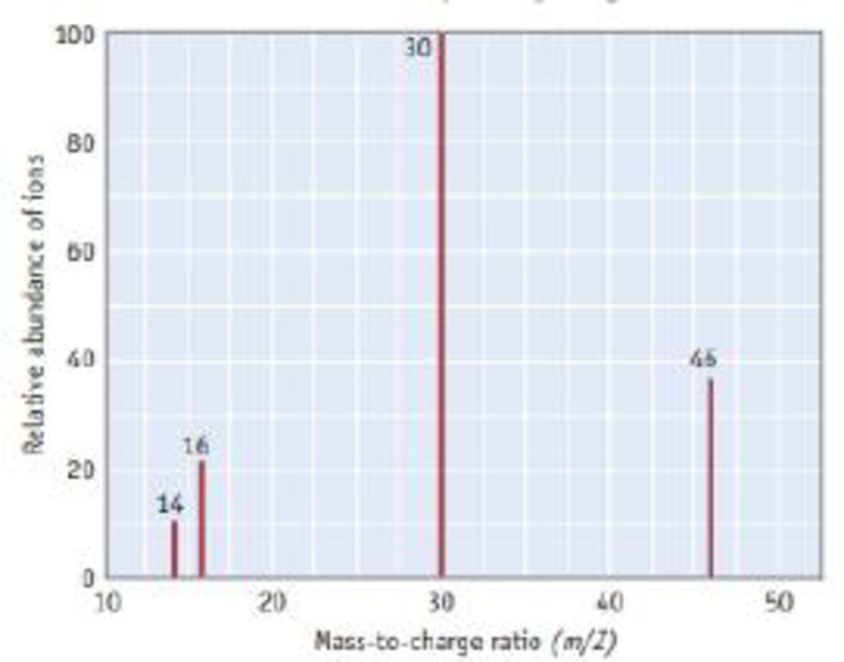Chapter 2, Problem 97PS

Chapter
Section
Textbook Problem

The mass spectrum of nitrogen dioxide is illustrated here. (a) Identify the cations present for each of the four peaks in the mass spectrum. (b) Does the mass spectrum provide evidence that the two oxygen atoms are attached to a central nitrogen atom (ONO), or that an oxygen atom is at the center (NOO)? Explain.(a)

Interpretation Introduction

Interpretation:

For the mass spectrum of nitrogen dioxide, the cations present for each of the four peaks has to be identified.

The bonding of oxygen atoms with nitrogen are needed to be explained.

Concept introduction:

Mass spectroscopy: It is a form of spectroscopic technique which is used for the elucidation of the molecules, depending upon the mass of the molecule.

Parent ion peak: It is defined as the prominent peak in the mass spectrum of the molecule which represents the not fragmented molecular ion in the given molecule with greater m/z value.

Base peak: It is the tallest peak in the spectrum.

NO2 ionize to form positive ions (NO2+ ). Some of them fragment into smaller ions (N+, O+ and NO+).

Explanation

The four peaks in the mass spectrum are 14, 16, 30 and 46.

The four ions produced by nitrogen dioxide are N+, O+, NO+ and NO2+

(b)

Interpretation Introduction

Interpretation:

The bonding of oxygen atoms with nitrogen are needed to be explained.

Concept introduction:

Mass spectroscopy: It is a form of spectroscopic technique which is used for the elucidation of the molecules, depending upon the mass of the molecule.

Parent ion peak: It is defined as the prominent peak in the mass spectrum of the molecule which represents the not fragmented molecular ion in the given molecule with greater m/z value.

Base peak: It is the tallest peak in the spectrum.

NO2 ionize to form positive ions (NO2+ ). Some of them fragment into smaller ions (N+, O+ and NO+).

Still sussing out bartleby?

Check out a sample textbook solution.

See a sample solution

The Solution to Your Study Problems

Bartleby provides explanations to thousands of textbook problems written by our experts, many with advanced degrees!

Get Started

Each gene is a blueprint that directs the production of one or more of the body's organs. T F

Nutrition: Concepts and Controversies - Standalone book (MindTap Course List)

What is the definition of science?

An Introduction to Physical Science

What is genomics?

Human Heredity: Principles and Issues (MindTap Course List)

30-39 Which vitamins contain a sulfur atom?

Introduction to General, Organic and Biochemistry

What is the structure and function of the cytoskeleton?

Biology: The Dynamic Science (MindTap Course List)